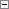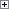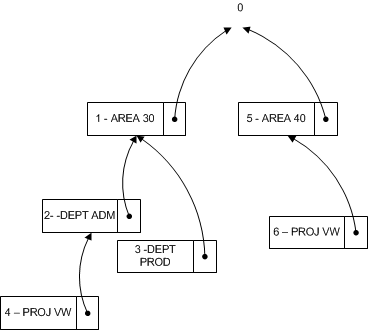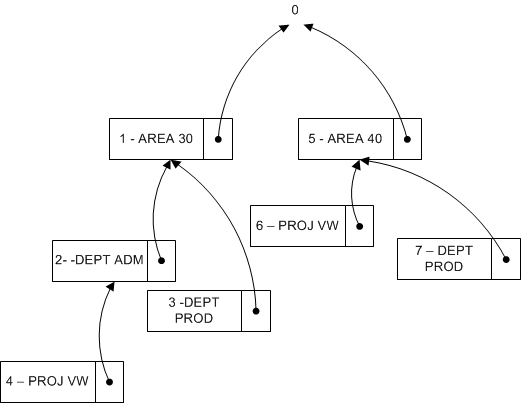When you close a window after you edit a set of dimensions, Microsoft Dynamics NAV evaluates whether the edited set of dimensions exists. If the set does not exist, a new set is created and the dimension combination ID is returned.

Building Search Tree

Table 481 Dimension Set Tree Node is used when Microsoft Dynamics NAV evaluates whether a set of dimensions already exists in table 480 Dimension Set Entry table. The evaluation is performed by recursively traversing the search tree starting at the top level numbered 0. The top level 0 represents a dimension set with no dimension set entries. The children of this dimension set represent dimension sets with only one dimension set entry. The children of these dimension sets represent dimension sets with two children, and so on.

Example 1

The following diagram represents a search tree with six dimension sets. Only the distinguishing dimension set entry is displayed in the diagram.The following table describes a complete list of dimension set entries that make up each dimension set.

Dimension Sets Dimension Set Entries

Set 0

None

Set 1

AREA 30

Set 2

AREA 30, DEPT ADM

Set 3

AREA 30, DEPT PROD

Set 4

AREA 30, DEPT ADM, PROJ VW

Set 5

AREA 40

Set 6

AREA 40, PROJ VW

Example 2

This example shows how Microsoft Dynamics NAV evaluates whether a dimension set that consists of the dimension set entries AREA 40, DEPT PROD exists.

First, Microsoft Dynamics NAV checks whether AREA 40 exists as a child of the parent ID 0. Microsoft Dynamics NAV finds a hit in the Dimension Set Tree Node table. The hit is marked as the dimension set 5 in the diagram. After Microsoft Dynamics NAV has found the hit, it continues by checking whether DEPT PROD exists as a child of the dimension set 5. Because this is not the case, Microsoft Dynamics NAV creates a new dimension set by using the unused ID 7. The new dimension set 7 contains the dimension set entries AREA 40 and DEPT PROD. Microsoft Dynamics NAV also updates the Dimension Set Tree Node table to make sure that the search tree looks like the following diagram. Thus dimension set 7 becomes a child of the dimension set 5.Finding Dimension Set ID

At a conceptual level, Parent ID, Dimension, and Dimension Value, in the search tree, are combined and used as the primary key because Microsoft Dynamics NAV traverses the tree in the same order as the dimension entries. The GET function (record) is used to search for dimension set ID. The following code example shows how to find the dimension set ID when there are three dimension values.Copy Code
DimSet."Parent ID" := 0;  // 'root'
IF UserDim.FINDSET THEN
REPEAT
DimSet.GET(DimSet."Parent ID",UserDim.DimCode,UserDim.DimValueCode);
UNTIL UserDim.NEXT = 0;
EXIT(DimSet.ID);

However, to preserve the ability of Microsoft Dynamics NAV to rename a dimension and dimension value, table 348 Dimension Value is extended with an integer field of Dimension Value ID. This table converts the field pair Dimension and Dimension Value to an integer value. When you rename the dimension and dimension value, the integer value is not changed.Copy Code
DimSet."Parent ID" := 0;  // 'root'
IF UserDim.FINDSET THEN
REPEAT
DimSet.GET(DimSet.ParentID,UserDim."Dimension Value ID");
UNTIL UserDim.NEXT = 0;
EXIT(DimSet.ID);